Courses

# Worksheet - Area and its Boundary Notes | EduRev

## Class 5 : Worksheet - Area and its Boundary Notes | EduRev

The document Worksheet - Area and its Boundary Notes | EduRev is a part of the Class 5 Course Mathematics for Class 5 (V) - CBSE and NCERT Curriculum.
All you need of Class 5 at this link: Class 5

Q.1. What is the area of this rectangular field?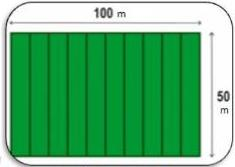Ans.
5000 m2

Q.2. Find the total boundary of the given piece A?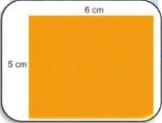Ans.
22 cm

Q.3. Find the perimeter of the given shape made up by the equilateral triangles?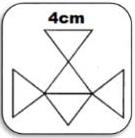Ans.
52 cm

Q.4. Calculate the area of this stamp.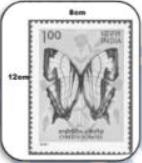Ans.
96 cm

Q.5. The fencing of a square garden is 120 cm long. How long is one side of the  garden?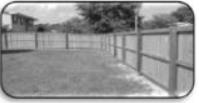Ans.
30cm

Q.6. What is the area of the green marked portion?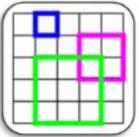Ans.
9 cm2

Q.7. In which unit you will measure a handkerchief?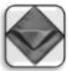Ans.
Square centimeters

Q.8. Which garden's area is bigger and by how much?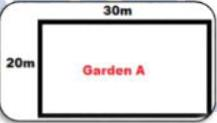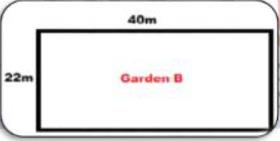Ans.
Garden B by 280m2.

Q.9. Can you find and compare the areas of both the rectangles?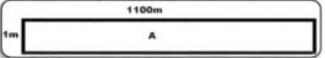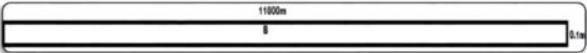Ans.
1100m2. They both are same.

Q.10. Can you find the missing variables?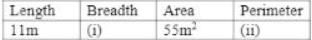Ans.
(ii) Perimeter = 32 m

Q.11. A thin wire 120 cm long is formed into a rectangle. If the length of this rectangle is 40 cm. what is its width?
Ans.
20 cm

Q.12. Find the boundary of this shape?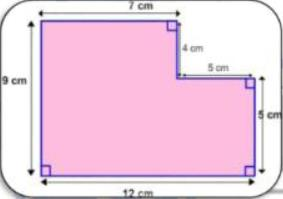Ans.
42cm

Q.13. Find the area of the given shape.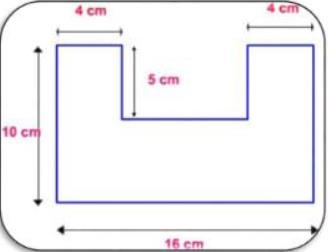Ans.
120 cm2

Q.14. Jack plans to tile his classroom floor with square tiles. Each side of the tile is 8 cm. His classroom is 160 cm in length and 240 cm wide. How many tiles will he need?
Ans.
600 tiles

Q.15. What is the perimeter of this given shape?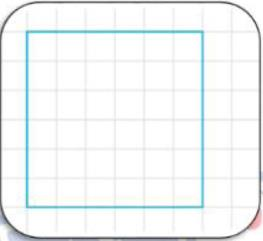Ans.
24 cm

Q.16. If you were to write the area of each of these, which column would you choose?

 Object Square cm Square m Square km (i) Total land of city (ii) Light pole

Ans. (i) Square km
(ii) Square m

Q.17. What is the area of the given shape in real life if each square unit 1 cm is 1 in in real life?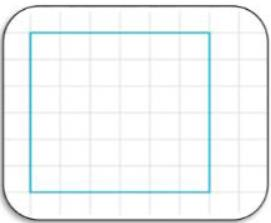Ans.
36 m2

Q.18. A greeting card is made up of thick paper sheet of length 32 cm and width 11 cm? What is its area?
Ans.
352 cm2

Q.19. Divya needs a long ribbon to decorate a card of length  80 cm and breadth 100 cm. How long ribbon will she need if she wants to put the ribbon along the a border of the card.
Ans.
360 cm

Q.20. Akhil plans to decorate his room wall with square charts. Each side of the chart is 20 cm. The wall is 320 cm in length and 180 cm wide. How many charts will he need?
Ans.
144 charts

Offer running on EduRev: Apply code STAYHOME200 to get INR 200 off on our premium plan EduRev Infinity!

## Mathematics for Class 5 (V) - CBSE and NCERT Curriculum

50 videos|43 docs|39 tests

,

,

,

,

,

,

,

,

,

,

,

,

,

,

,

,

,

,

,

,

,

;